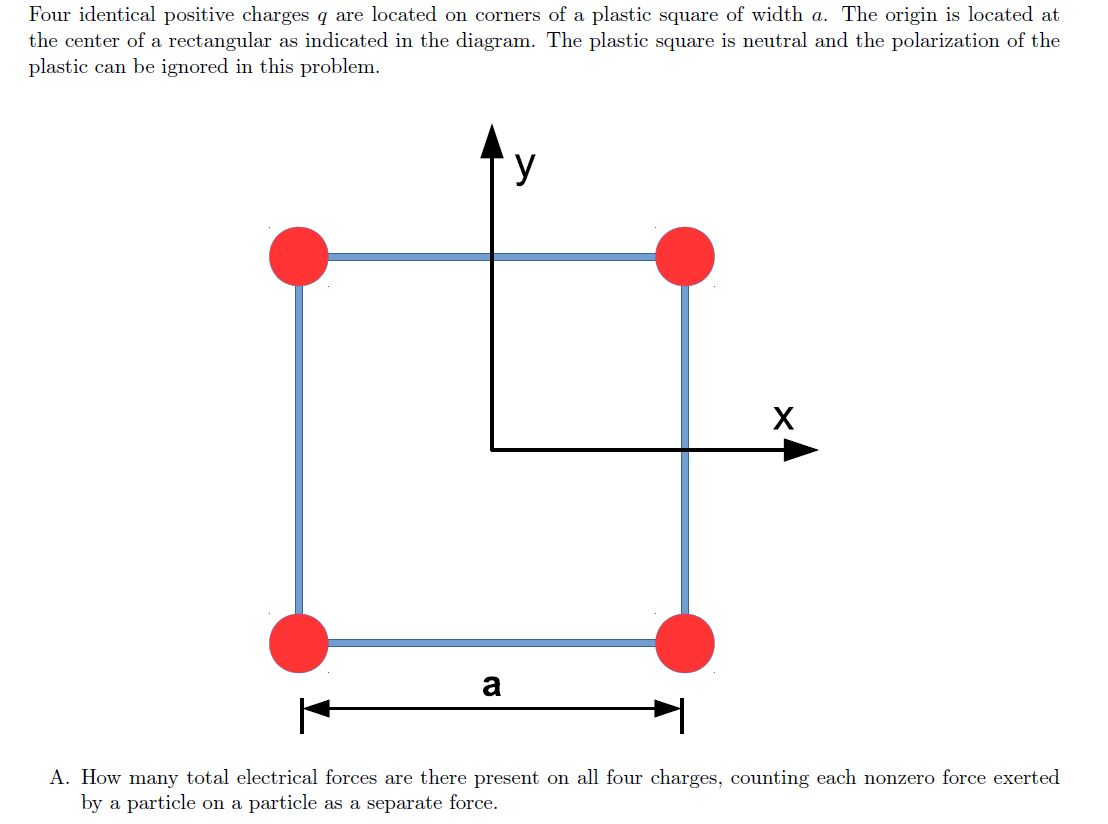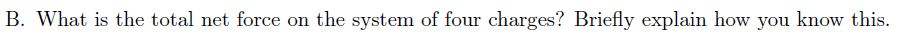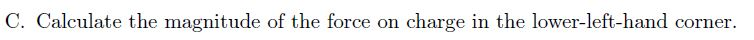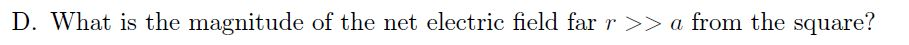# Question Solved1 AnswerFour identical positive charges q are located on corners of a plastic square of width a. The origin is located at the center of a rectangular as indicated in the diagram. The plastic square is neutral and the polarization of the plastic can be ignored in this problem. У X a A. How many total electrical forces are there present on all four charges, counting each nonzero force exerted by a particle on a particle as a separate force. B. What is the total net force on the system of four charges? Briefly explain how you know this. C. Calculate the magnitude of the force on charge in the lower-left-hand corner. D. What is the magnitude of the net electric field far r >> a from the square?Transcribed Image Text: Four identical positive charges q are located on corners of a plastic square of width a. The origin is located at the center of a rectangular as indicated in the diagram. The plastic square is neutral and the polarization of the plastic can be ignored in this problem. У X a A. How many total electrical forces are there present on all four charges, counting each nonzero force exerted by a particle on a particle as a separate force. B. What is the total net force on the system of four charges? Briefly explain how you know this. C. Calculate the magnitude of the force on charge in the lower-left-hand corner. D. What is the magnitude of the net electric field far r >> a from the square?
More
Transcribed Image Text: Four identical positive charges q are located on corners of a plastic square of width a. The origin is located at the center of a rectangular as indicated in the diagram. The plastic square is neutral and the polarization of the plastic can be ignored in this problem. У X a A. How many total electrical forces are there present on all four charges, counting each nonzero force exerted by a particle on a particle as a separate force. B. What is the total net force on the system of four charges? Briefly explain how you know this. C. Calculate the magnitude of the force on charge in the lower-left-hand corner. D. What is the magnitude of the net electric field far r >> a from the square?